CAT  >  Advice to Aspirants - CAT : LR DI

# Advice to Aspirants - CAT : LR DI Notes - CAT

## Document Description: Advice to Aspirants - CAT : LR DI for CAT 2022 is part of CAT preparation. The notes and questions for Advice to Aspirants - CAT : LR DI have been prepared according to the CAT exam syllabus. Information about Advice to Aspirants - CAT : LR DI covers topics like and Advice to Aspirants - CAT : LR DI Example, for CAT 2022 Exam. Find important definitions, questions, notes, meanings, examples, exercises and tests below for Advice to Aspirants - CAT : LR DI.

Introduction of Advice to Aspirants - CAT : LR DI in English is available as part of our CAT preparation & Advice to Aspirants - CAT : LR DI in Hindi for CAT courses. Download more important topics, notes, lectures and mock test series for CAT Exam by signing up for free. CAT: Advice to Aspirants - CAT : LR DI Notes - CAT
 1 Crore+ students have signed up on EduRev. Have you?

Advice to Aspirants: (CAT)

Cracking the Common Admission Test (CAT) is not only about perseverance and hard-work. You also need to think strategically about the right choices to make it count. In this era of competition, one has to strike a perfect balance between smart work and hard-work. So, keeping that in mind, we and you, together will devise the perfect strategy, which will save tonnes of your time and ensure that you get a head-start in the race.

Purpose of this document is to make you understand how to study for the CAT exam. By the end of this document, you will have clarity on how to tackle every topic that is included in the syllabus for the exam. We, at EduRev, believe in 3R - Read, Revise and Repeat and strongly recommend you to use this strategy throughout your preparation for this exam.

How and where to start?

Before starting any subject, it is mandatory that you go through the syllabus first. We suggest you get familiar with the syllabus as having the syllabus at your fingertips is 20% of the preparation done. You can check the syllabus here:

Once you are familiar with the syllabus, we suggest you go through the previous year's questions to get an idea about the type and level of the questions being asked in the Exam. You can access previous year questions with solutions and  detailed explanations here (add link).

Here are a few Questions to help you understand the type of questions asked during the exam:

Ques 1 (CAT 2020) : A farmer had a rectangular land containing 205 trees. He distributed that land among his four daughters – Abha, Bina, Chitra and Dipti by dividing the land into twelve plots along three rows (X,Y,Z) and four Columns (1,2,3,4) as shown in the figure below: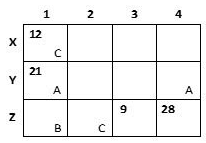The plots in rows X, Y, Z contained mango, teak and pine trees respectively. Each plot had trees in non-zero multiples of 3 or 4 and none of the plots had the same number of trees. Each daughter got an even number of plots. In the figure, the number mentioned in top left corner of a plot is the number of trees in that plot, while the letter in the bottom right corner is the first letter of the name of the daughter who got that plot (For example, Abha got the plot in row Y and column 1 containing 21 trees). Some information in the figure got erased, but the following is known:

1. Abha got 20 trees more than Chitra but 6 trees less than Dipti.
2. The largest number of trees in a plot was 32, but it was not with Abha.
3. The number of teak trees in Column 3 was double of that in Column 2 but was half of that in Column 4.
4. Both Abha and Bina got a higher number of plots than Dipti.
5. Only Bina, Chitra and Dipti got corner plots.
6. Dipti got two adjoining plots in the same row.
7. Bina was the only one who got a plot in each row and each column.
8. Chitra and Dipti did not get plots which were adjacent to each other (either in row / column / diagonal).
9. The number of mango trees was double the number of teak trees.

Q. How many mango trees were there in total?
A. 126
B. 84
C. 98
D. 49

Q. Which of the following is the correct sequence of trees received by Abha, Bina, Chitra and Dipti in that order?

A. 50, 69, 30, 56
B. 60, 39, 40, 66

C. 44, 87, 24, 50
D. 54, 57, 34, 60

Q. How many pine trees did Chitra receive?

A. 15

B. 18

C. 30

D. 21

Q. Who got the plot with the smallest number of trees and how many trees did that plot have?

A. Abha, 4 trees

B. Dipti, 6 trees

C. Bina, 3 trees

D. Bina, 4 trees

Q. Which of the following statements is NOT true?

A. Bina got 32 pine trees.

B. Chitra got 12 mango trees.

C. Abha got 41 teak trees.

D. Dipti got 56 mango trees.

Q. Which column had the highest number of trees?

A. 3

B. Cannot be determined

C. 2

D. 4

Explanation:
From the statement point 4) and 6), we can say both Abha and Bina will get 4 plots each (as each daughter got an even number of plots) .

As Dipti got 2 plots, which also means Chitra gets 2 plots (12- 4-4-2 = 2).

Now let's say Abha gets “a” trees.

So number of trees with Chitra = a – 20

And number of trees with Dipti = a + 6

Now if the number of teak trees in column 2 was x then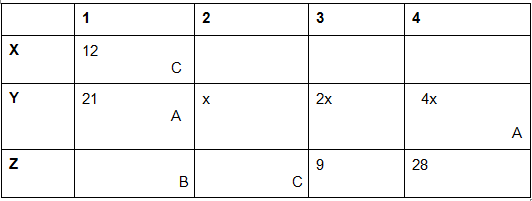Total number of teak trees = x + 2x + 4x + 21 = 7x + 21
From statement point 9) number of mango trees = 2(7x+21 ) = 14x +42
Now as we can see minimum number of trees in Row Z – column 1 and column 2 can be 7 (3, 4 or 4,3)
Which means 7x+21 + 14x + 42 <= 205 - (7+9+28) = 161
Or 21x <= 98
As x is an integer and multiple of 3 or 4 so x can be either 3 or 4 only ( if x = 5 , 21x = 105 > 98)
If x = 3, not possible because if x = 3 , 4x = 12 which means two plots (Row X column 1 and Row Y column 4) will have the same number of trees, which is not possible.

Thus, x = 4.
Number of teak trees = 7x + 21 = 7*4 + 21 = 49
Number of mango trees = 14x + 42 = 14*4 + 42 = 98
Thus Number of pine trees = 205 – 98 – 49 = 58
Sum of number of trees in Row Z – column 1 and column 2 = 58 – 28 – 9 = 21
Possible pair (3,18) or (6,15)
From the statement point 5) and 6) Dipti must get 2 plots one each in column 3 and column 4. So she can get a plot only in Row X or Row Z.

Now from point 8) Dipti didn’t get a plot adjacent to Chitra so Dipti should get plots in row X.
Thus we can make the following arrangements –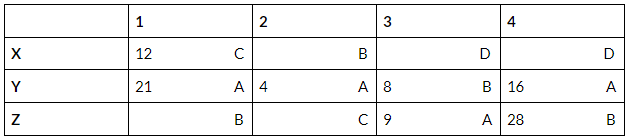Number of trees with A = 9+21+ 16 +4 = 50

So a = 50

Now number of trees with C = a -20 = 50 – 20 = 30

Number of trees with D= a+6 = 50+6 =56

Number of trees with B = 205 - (56 + 50 + 30) = 69

So, number of trees in Row Z column 2 = 30 – 12 = 18

Number of trees in Row Z column 1 = 21 – 18 = 3

Number of trees in Row X column 2 = 69 – 3 – 8 – 28 = 30

D has the plot with 32 trees.

Thus final trees – plot diagram will be as below –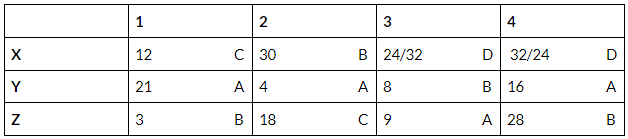All the questions can be answered now.

Problem mostly faced by aspirants like you:

Practice questions are either too easy, or are too tough that the first step is very difficult to figure out. It is very important to understand the thought process of someone who scores well in the section. So, understanding the thought process of an expert while solving a CAT - level DILR question set is what will help you bridge the gap and get to 99 percentile.

Lakshya Kumar, CAT 2019 topper (99.99 percentile) shared his preparation strategy for CAT DILR sections.

Lakshya stated - “Data Interpretation and Logical Reasoning section during my preparation phase was attempting a wide variety of questions from the previous year papers of CAT and then re-attempting them. During the mocks, I made sure that I read all the question sets fire and then picked out the ones that were easy and could be managed instead of doing the questions in order of question paper. After all, CAT is never about attempting all questions but getting one more right than your competitors”.

Key Takeaway:

Selecting the right puzzle is half the battle won.”

Focus enormously on finding the right puzzle in an exam setting. Make sure, you definitely attempt the right puzzles, not the toughest ones.

Weightage of Data Interpretation and Logical Reasoning:

• There is a 40 minutes time limit for each section. Each correct answer is awarded 3 marks and each wrong answer carries a penalty of -1 mark. For non-MCQs there is no negative marking.
• Data Interpretation and Logical Reasoning- 12 questions from Data Interpretation and 8 questions on Logical Reasoning.

Data Interpretation and Logical Reasoning:

It is considered to be the most challenging section. This section assesses your ability to solve problem-solving skills. Regular practice will help you to get an edge over others. You should begin with understanding and developing basic concepts. The various topics included in this section are:

• Word Problems
• Graphs
• Sequencing
• Grid Puzzles
• Maths Puzzles

Some tips that will help you to score well in CAT 2021 Verbal Ability and Reading Comprehension section:

• Crack the Logic behind the data. Questions of this section are all about hidden information in the question. Don’t limit yourself to the questions in the study material.
• Use your leisure time by solving Sudoku and puzzles. You can also  enhance your skills by playing mobile games like 2048. Such activities help in brainstorming.
• Work with brain teasers to improve your logical reasoning for CAT and make it fun.
• In this section, solving a certain number of questions with the utmost accuracy will suffice to get a good percentage in this section. (Attempt only if you know it is correct)
• Give equal importance to the information provided in the question and the factual data.
• Solve previous year papers of CAT to get a gist to types of questions (Add link to previous year questions). You can also solve previous year papers as tests here (add link).
• Take mock tests on a regular basis to gauge the preparation level (Add link to mock tests)
• CAT is a timed test – So make sure that you solve questions quickly. Here is a link for shortcut and trick videos (Add link to videos).

Structure of this subject on EduRev:

EduRev Videos
First watch the EduRev videos recommended for your basic understanding.

We provide videos that explain basic concepts of every topic, tell you how to solve questions and also identify the basic logic behind the Problems.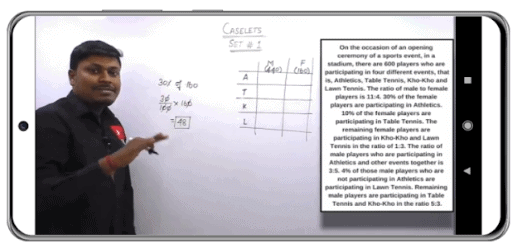How to deal Caselets Question

EduRev Notes
Once you have seen the content of the videos, you would need notes to understand and revise the topics and EduRev has made them for you.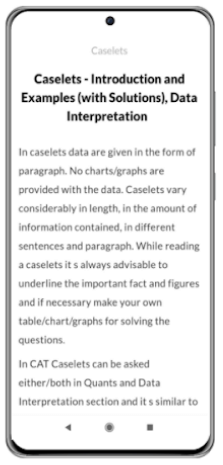We provide notes for every topic which contain theory, solved examples and intext questions with proper explanation.

EduRev Tests
We have made quick tests along with the detailed notes and video lectures to help you revise the topic just after you've studied it. These tests help you check your knowledge instantly and are a great self-improvement/instant feedback tool.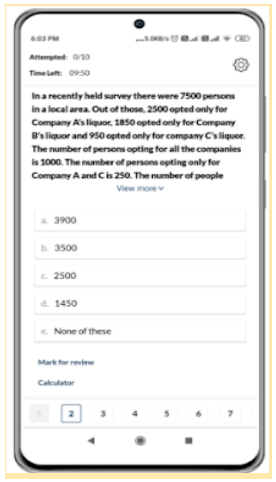Other Features

• Analysis of what you have learnt
• Detailed solutions for each question
• Nationwide rankings
• Mock Tests

Previous Year Papers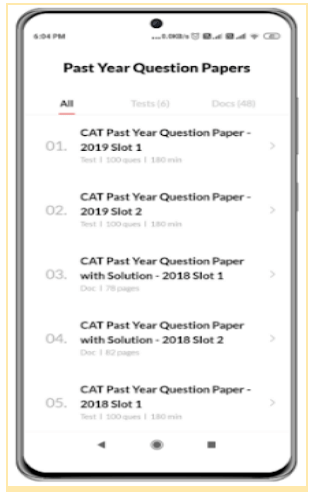Which books to read?
• Data Interpretation and Logical Reasoning:
(i) How to Prepare for Logical Reasoning for the CAT and How to Prepare for Data Interpretation for the CAT by Arun Sharma,
(ii) Logical Reasoning and Data Interpretation for the CAT by Nishit K. Sinha
(iii) Data Interpretation & Data Sufficiency by Ananta Ashisha

We, at EduRev, are always available to make the lives of the students’ easier. We believe in revolutionising the Education so that it becomes a more lively experience for you.

WE, here at EduRev, are working tirelessly to make that happen.

The document Advice to Aspirants - CAT : LR DI Notes - CAT is a part of CAT category.
All you need of CAT at this link: CAT
 Use Code STAYHOME200 and get INR 200 additional OFF

### How to Prepare for CAT

Read our guide to prepare for CAT which is created by Toppers & the best Teachers

Track your progress, build streaks, highlight & save important lessons and more!

,

,

,

,

,

,

,

,

,

,

,

,

,

,

,

,

,

,

,

,

,

;